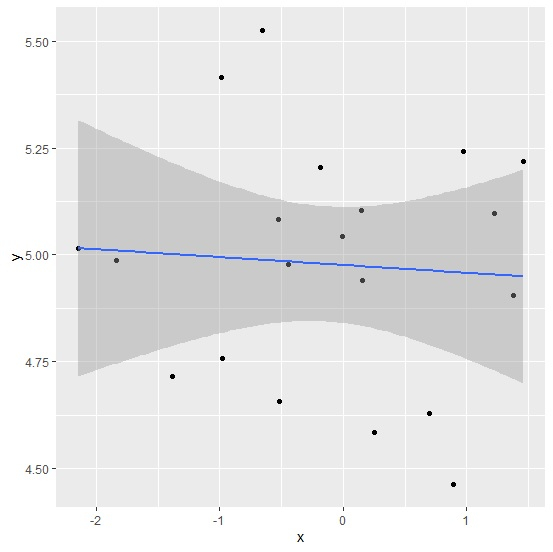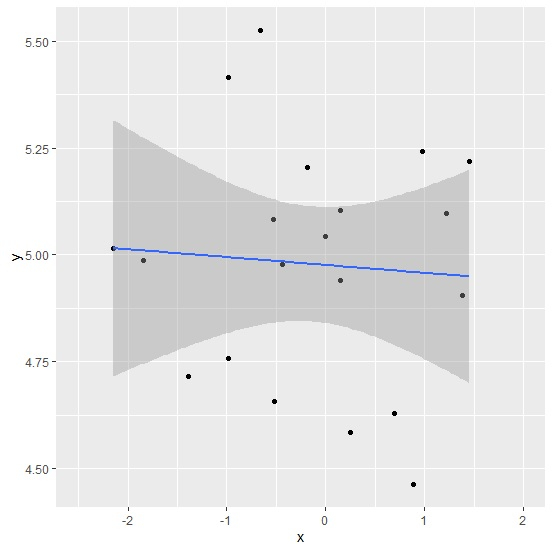# How to limit the length of regression line using ggplot2 in R?

To limit the length of regression line using ggplot2 in R, we can follow the below steps −

• First of all, create a data frame.
• Then, create the scatterplot using ggplot2 with regression line.
• After that, create the scatterplot with regression and add xlim function.

## Create the data frame

Let's create a data frame as shown below −

Live Demo

x<-rnorm(20)
y<-rnorm(20,5,0.24)
df<-data.frame(x,y)
df

On executing, the above script generates the below output(this output will vary on your system due to randomization) −

      x          y
1 -0.988803542 5.414778
2 0.698684062 4.627536
3 0.891143869 4.461881
4 -0.516950077 4.657352
5 0.145037494 5.105152
6 -0.528889920 5.083324
7 1.379575319 4.904177
8 0.975201898 5.240997
9 -0.983342263 4.757083
10 1.458508452 5.219206
11 0.151370004 4.941212
12 -0.656576442 5.526522
13 1.221435134 5.096742
14 -0.441853461 4.976549
15 -0.007734295 5.042228
16 0.250223974 4.584807
17 -1.839967551 4.985860
18 -1.386944529 4.716434
19 -2.143977782 5.016142
20 -0.187381571 5.205459

## Create the scatterplot with regression line

Using geom_point and stat_smooth function of ggplot2 package to create the scatterplot with regression line −

x<-rnorm(20)
y<-rnorm(20,5,0.24)
df<-data.frame(x,y)
library(ggplot2)
ggplot(df,aes(x,y))+geom_point()+stat_smooth(method="lm")
geom_smooth() using formula 'y ~ x'

### Output## Create the limited regression line

Adding xlim function to the above function to create the limited regression line −

x<-rnorm(20)
y<-rnorm(20,5,0.24)
df<-data.frame(x,y)
library(ggplot2)
ggplot(df,aes(x,y))+geom_point()+stat_smooth(method="lm")+xlim(-2.5,2)
geom_smooth() using formula 'y ~ x'

### Output